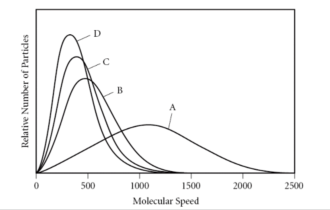# Problem: Which of the gases in the graph below has the largest molar mass?A) AB) BC) CD) DE) There is not enough information to determine.

###### FREE Expert Solution
87% (159 ratings)
###### FREE Expert Solution

We’re being asked which gas has the largest molar mass at 25 ˚C.

In the graph, the y-axis is the relative number of particles while the x-axis is the molecular speed. We can think of the molar mass of a gas as being related to the number of particles ( molar mass =  particles).

Recall that the molecular speed of a gas is given by:where R = gas constant, T = temperature, and M = molar mass of the gas.

87% (159 ratings)###### Problem Details

Which of the gases in the graph below has the largest molar mass?

A) A

B) B

C) C

D) D

E) There is not enough information to determine.What scientific concept do you need to know in order to solve this problem?

Our tutors have indicated that to solve this problem you will need to apply the Kinetic Molecular Theory concept. You can view video lessons to learn Kinetic Molecular Theory. Or if you need more Kinetic Molecular Theory practice, you can also practice Kinetic Molecular Theory practice problems.

What is the difficulty of this problem?

Our tutors rated the difficulty ofWhich of the gases in the graph below has the largest molar ...as low difficulty.

How long does this problem take to solve?

Our expert Chemistry tutor, Sabrina took 2 minutes and 7 seconds to solve this problem. You can follow their steps in the video explanation above.

What professor is this problem relevant for?

Based on our data, we think this problem is relevant for Professor Benson's class at MONTGOMERY COLLEGE.